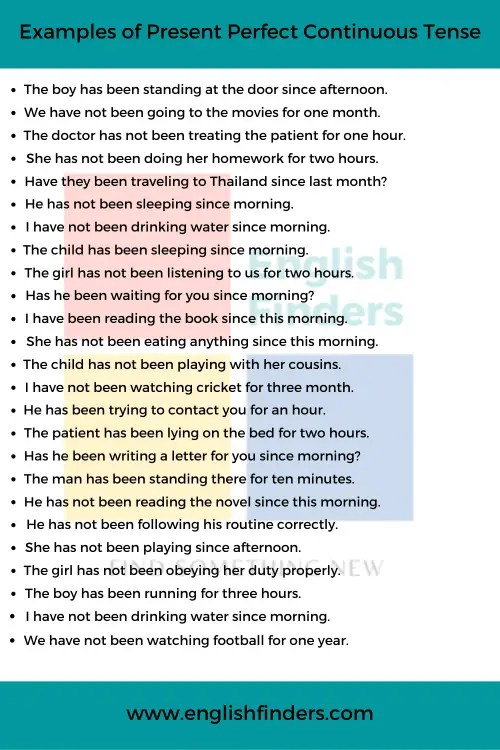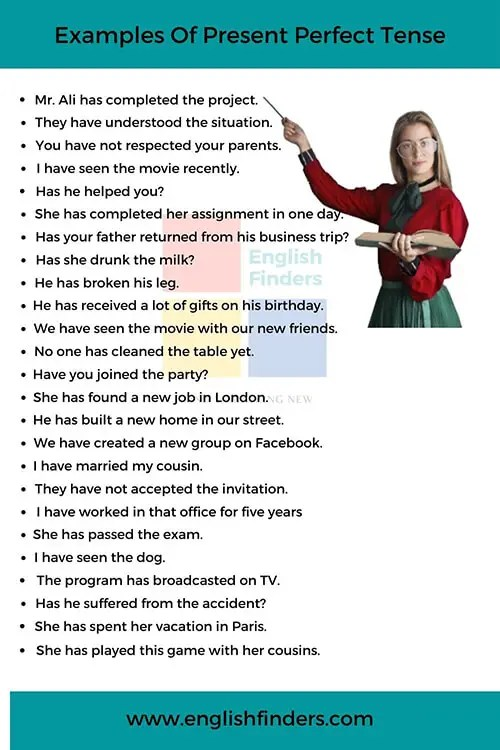# Tenses## 50 Examples of Present Perfect Continuous Tense

Present Perfect Continuous is one of the four tenses in the present tense. It indicates that something started in the past and is still continuing now. Present perfect continuous tense takes “has been” “have been” as its helping verb and (ing) is added after the main verb. 50 Examples of Present Perfect Continuous Tense Let’s …## 50 Examples Of Present Perfect Tense

This lesson will discuss the 50 useful examples of the present perfect tense. Present perfect tense describes an action that has been finished but its consequence still available. It takes “have” “has” as its helping verb, and the past participle form is added after the main verb. 50 Examples Of Present Perfect Tense Let’s see …## 50 Examples of Present Continuous Tense

This lesson will discuss the 50 useful examples of present continuous tense. The present continuous tense is used to indicate an action that is happening now. In other words, we can say the Present continuous tense is used to describe an ongoing action. It is formed by using the “be” verbs (am, is, are) followed …## 50 Useful Examples of Simple Present Tense

In this lesson, we will discuss the 50 useful examples of simple present tense. Simple Present Tense or Present Indefinite Tense indicates a present action or habitual fact. The third-person singular number has ‘s’ or ‘es’ at the end of its main verb. Let’s see some examples of the simple present tense: Rakin reads his …## Future Continuous Tense | Definition, Structure, and Examples

Future Continuous Tense Future Continuous Tense indicates an action that will occur in the future and continue for some time. Let us see some examples of Future Continuous Tense to get a clear conception about it: I will be running the program. He will be taking the challenge. You will be doing a great job. …## Future Indefinite Tense | Definition, Structure, and Examples

Future Indefinite Tense We need to use Future Indefinite Tense to indicate any future action. The definition of Future Indefinite Tense is easy. Future Indefinite Tense describes an action that will be occurred in the future. Let us see some examples of Future Indefinite Tense to get a clear conception about it: I will meet …